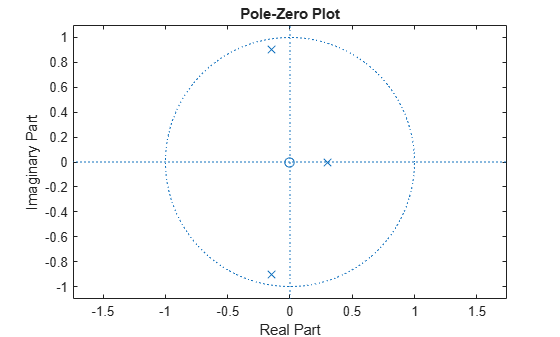# eqtflength

Equalize lengths of transfer function numerator and denominator

## Syntax

``[b,a] = eqtflength(num,den)``
``[b,a,n,m] = eqtflength(num,den)``

## Description

````[b,a] = eqtflength(num,den)` modifies the vector `num` or the vector `den` so that the resulting output vectors `b` and `a` have the same length. `b` and `a` represent the same discrete-time transfer function as `num` and `den`, but are of equal length.```

example

````[b,a,n,m] = eqtflength(num,den)` returns the numerator order `n` and the denominator order `m`, not including any trailing zeros.```

## Examples

collapse all

Consider the following discrete-time SISO transfer function model:

$H\left(z\right)=\frac{2{z}^{-2}}{4+3{z}^{-2}-{z}^{-3}}$.

Equalize the numerator and denominator polynomial lengths. Determine the polynomial orders.

```num = [0 0 2]; den = [4 0 3 -1]; [b,a,n,m] = eqtflength(num,den)```
```b = 1×4 0 0 2 0 ```
```a = 1×4 4 0 3 -1 ```
```n = 2 ```
```m = 3 ```

Visualize the poles and zeros of the transfer function.

`zplane(b,a)`Convert the transfer function with equalized numerator and denominator to state-space form. `b` and `a` must have equal lengths to find the state-space representation of the discrete-time transfer function.

`[A,B,C,D] = tf2ss(b,a)`
```A = 3×3 0 -0.7500 0.2500 1.0000 0 0 0 1.0000 0 ```
```B = 3×1 1 0 0 ```
```C = 1×3 0 0.5000 0 ```
```D = 0 ```

## Input Arguments

collapse all

Numerator polynomial coefficients of discrete-time transfer function, specified as a vector.

Data Types: `double`
Complex Number Support: Yes

Denominator polynomial coefficients of discrete-time transfer function, specified as a vector.

Data Types: `double`
Complex Number Support: Yes

## Output Arguments

collapse all

Numerator polynomial coefficients of discrete-time transfer function, returned as a row vector. `b` has the same length as `a`.

Denominator polynomial coefficients of discrete-time transfer function, returned as a row vector. `a` has the same length as `b`.

Numerator order, returned as an integer. Any trailing zeros in `b` are excluded when computing `n`.

Denominator order, returned as an integer. Any trailing zeros in `a` are excluded when computing `m`.

## Tips

• Use `eqtflength` to obtain a numerator and denominator of equal length before applying transfer function conversion functions such as `tf2ss` and `tf2zp` to discrete-time models.

## Algorithms

`eqtflength(num,den)` appends zeros to either `num` or `den` as necessary. `eqtflength` removes any trailing zeros that `num` and `den` have in common.

## Version History

Introduced before R2006a